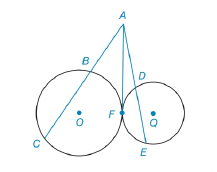Chapter 6.3, Problem 27EElementary Geometry For College St...

7th Edition
Alexander + 2 others
ISBN: 9781337614085

Solutions

Chapter
SectionElementary Geometry For College St...

7th Edition
Alexander + 2 others
ISBN: 9781337614085
Textbook Problem

In Exercises 27 to 30, provide a paragraph proof.Given: ⊙ O     a n d     ⊙ Q are tangent at point FSecant A C   ¯   t o     ⊙ O Secant A E   ¯   t o     ⊙ Q Common internal tangent A F ¯ Prove: A C ⋅ A B = A E ⋅ A DTo determine

To find:

Explanation

Given that, OandQ are tangent at point F, Secant AC¯toO, Secant AE¯toQ.

The diagrammatic representation is given below,

Theorem:

If a tangent segment and a secant segment are drawn to a circle from an external point, then the square of the length of the tangent equals the product of the length of the secant with the length of its external segment.

By using the theorem for the circle O to get the following,

AF2=ACAB

Still sussing out bartleby?

Check out a sample textbook solution.

See a sample solution

The Solution to Your Study Problems

Bartleby provides explanations to thousands of textbook problems written by our experts, many with advanced degrees!

Get Started

Evaluate the expression sin Exercises 116. 3(2)0

Finite Mathematics and Applied Calculus (MindTap Course List)

Use series to evaluate the limit. 64. limx01+x112xx2

Single Variable Calculus: Early Transcendentals

True or False: If f(c) exists and f has a local minimum at c, then f(c) = 0.

Study Guide for Stewart's Single Variable Calculus: Early Transcendentals, 8th

π does not exist

Study Guide for Stewart's Multivariable Calculus, 8th